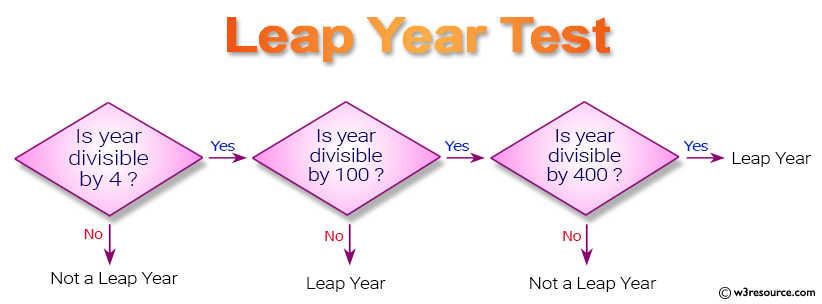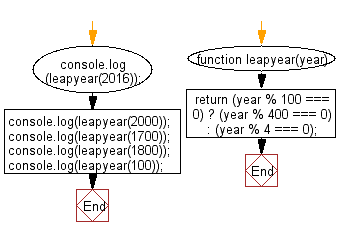# JavaScript: Check whether a given year is a leap year in the Gregorian calendar

## JavaScript Basic: Exercise-6 with Solution

Write a JavaScript program to determine whether a given year is a leap year in the Gregorian calendar.

Pictorial Presentation:Sample Solution-1:

JavaScript Code:

``````function leapyear(year)
{
return (year % 100 === 0) ? (year % 400 === 0) : (year % 4 === 0);
}
console.log(leapyear(2016));
console.log(leapyear(2000));
console.log(leapyear(1700));
console.log(leapyear(1800));
console.log(leapyear(100));
```
```

Sample Output:

```true
true
false
false
false
```

Explanation:

A leap year is a year containing one additional day added to keep the calendar year synchronized with the astronomical or seasonal year. Because seasons and astronomical events do not repeat in a whole number of days, calendars that have the same number of days in each year drift over time with respect to the event that the year is supposed to track. By inserting an additional day or month into the year, the drift can be corrected. A year that is not a leap year is called a common year.
Every year that is exactly divisible by four is a leap year, except for years that are exactly divisible by 100, but these centurial years are leap years if they are exactly divisible by 400. For example, the years 1700, 1800, and 1900 are not leap years, but the year 2000 is.

To determine whether a year is a leap year, follow these steps :

Step-1 : If the year is evenly divisible by 4, go to step 2. Otherwise, go to step 5.
Step-2 : If the year is evenly divisible by 100, go to step 3. Otherwise, go to step 4.
Step-3 : If the year is evenly divisible by 400, go to step 4. Otherwise, go to step 5.
Step-4 : The year is a leap year (it has 366 days).
Step-5 : The year is not a leap year (it has 365 days).

Flowchart:ES6 Version:

``````function leapyear(year) {
return year % 100 === 0 ? year % 400 === 0 : year % 4 === 0;
}
console.log(leapyear(2016));
console.log(leapyear(2000));
console.log(leapyear(1700));
console.log(leapyear(1800));
console.log(leapyear(100));
``````

Sample Solution-2:

• Use new Date(), setting the date to February 29th of the given year.
• Use Date.prototype.getMonth() to check if the month is equal to 1.

JavaScript Code:

``````const is_leapyear = year => new Date(year, 1, 29).getMonth() === 1;
console.log(is_leapyear(2016));
console.log(is_leapyear(2000));
console.log(is_leapyear(1700));
console.log(is_leapyear(1800));
console.log(is_leapyear(100));
```
```

Sample Output:

```true
true
false
false
false
```

Live Demo:

See the Pen JavaScript: Find Leap Year - basic-ex-6 by w3resource (@w3resource) on CodePen.

Improve this sample solution and post your code through Disqus

What is the difficulty level of this exercise?

﻿

## JavaScript: Tips of the Day

Set object

```const set = new Set([1, 1, 2, 3, 4]);

console.log(set);
```

The Set object is a collection of unique values: a value can only occur once in a set.
We passed the iterable [1, 1, 2, 3, 4] with a duplicate value 1. Since we cannot have two of the same values in a set, one of them is removed. This results in {1, 2, 3, 4}.

Ref: https://bit.ly/323Y0P6